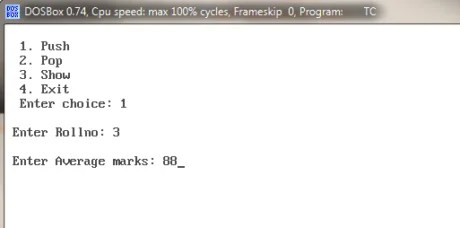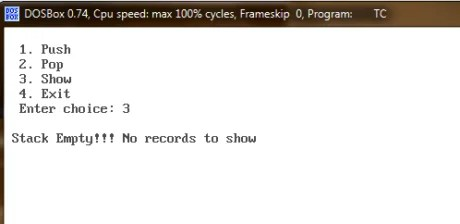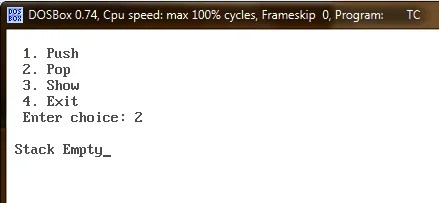Select Page

## Stacks using Arrays and Classes

In CBSE Class XII Computer Science using C++ board exams a question might come using stacks of one of the following types:

1. Stack implementation using Arrays (chances are less)
2. Stack implementation using Arrays of Structure and Classes. (Important)
3. Stack implementation using Linked Lists (Important)

### Correct way of implementing Stacks

This post is demonstrating the correct way of implementing Stacks using Arrays of Structure and Classes. Walk through the entire post – you will find:

• Complete solution keeping in mind CBSE syllabus
• Explanation of the code

### Explanation – Post-mortem of the code

Before we jump to the entire code, lets discuss few important points worth discussing:

• Program defines the constant variable named size and later use to describe the size of the array named stack used as a stack.
```// size once defined as constant can be used to describe the size of array
#define size 10```
• Using a constant variable is not necessary, from academics point of view. (If we don’t use it we will have to use the integer constant number as a upper limit where ever required.)
• Program uses structure named stud to hold actual data.
• Program uses a class named STK. Inside this class we create an array of the structure (stud) named stack. While specifying its size, we are using constant defined above – size. If we don’t want to use constant variable – just replace all the occurrences of constant variable size with 10.
```//Structure to hold actual data
struct stud
{
int roll;
int avgmarks;
};

class STK
{
stud stack[size];  // Array of structure, will behave like a stack
int top;
public:
STK();
void push();
void pop();
void show();
};```
• Special variable top is defined inside a class, which will always hold the index value of the top most element in the array named stack
• I must initially hold the value -1, when stack is empty.
• Since you can’t initialize the class variable while declaring it, a constructor function is employed to initialize the top with the value -1, moment the class object is created inside main() function.
```class STK
{
stud stack[size];
int top;             // variable top defined.
// int top=-1          // Wrong. It is not allowed to initialize a variable here.
public:
STK();               // Forward declaration of constructor
void push();
void pop();
void show();
};

//Constructor body
STK::STK()
{
top=-1;
}```
• Other functions push(), pop() and show() are self explanatory.  Still it is explained through internal comments, where ever I found necessary.
• Function main() is also provided so that you are able to run the entire program. Though in exam you will be asked to implement push(), pop() functions only. In a rare case though you might be asked to implement other parts as well.
• Full listing of the working program is listed below.

### Complete Program – Stack using Arrays

```#include<iostream.h>
#include<conio.h>

#define size 10

struct stud
{
int roll;
int avgmarks;
};

class STK
{
stud stack[size];
int top;
public:
STK();
void push();
void pop();
void show();
};

STK::STK()
{
top=-1;
}

void STK::push()
{
if(top<size)
{
int r,avgm;
cout<<"\n Enter Rollno: ";
cin>>r;
cout<<"\n Enter Average marks: ";
cin>>avgm;
top++;
stack[top].roll=r;
stack[top].avgmarks=avgm;
}
else
{
cout<<"\n Stack Full";
getch();
}
}

void STK::pop()
{
int r,avgm;
if(top==-1)
{
cout<<"\n Stack Empty";
}
else
{
r=stack[top].roll;
avgm=stack[top].avgmarks;
top--;
cout<<"\n Record popped (Roll,Average Marks): "<<r<<","<<avgm;
}
getch();
}
void STK::show()
{
if(top==-1)
{
cout<<"\n Stack Empty!!! No records to show";
}
else
{
for(int i=top;i>=0;i--)
{
cout<<"\n Rollno: "<<stack[i].roll<<", Average Marks: "<<stack[i].avgmarks;
cout<<"\n ______________________________________";
}
}
getch();
}

void main()
{
STK stk;
int choice=0;
while(choice!=4)
{
clrscr();
cout<<"\n  1. Push";
cout<<"\n  2. Pop";
cout<<"\n  3. Show";
cout<<"\n  4. Exit";
cout<<"\n  Enter choice: ";
cin>>choice;

if(choice==1)
{
stk.push();
}
else if(choice==2)
{
stk.pop();
}
else if(choice==3)
{
stk.show();
}
}
}```

### Output Screen shots – Stacks implementation using array of Structure and classes

• Pushing elements to a stack• Displaying the stack• Popping the top most element in the stack.• Displaying the stack after popping the element

###• Displaying stack after popping all the elements in a stack

###• Trying to pop the element when stack is empty.

###